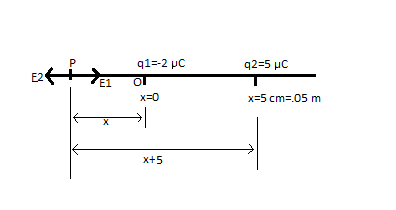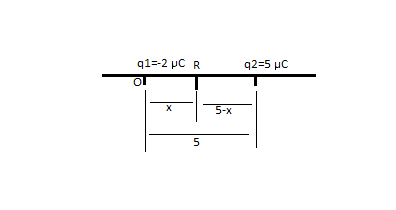# Two point charges, 5.0 \muC and -2.0\muC, are placed 5.0 cm apart on the x axis, such that the...

## Question:

Two point charges, 5.0 {eq}\mu {/eq}C and -2.0{eq}\mu {/eq}C, are placed 5.0 cm apart on the x axis, such that the -2.0 {eq}\mu {/eq}C charge is at x = 0 and the 5.0 {eq}\mu {/eq}C charge is at x = 5.0 cm.

(a) At what point(s) along the x axis is the electric field zero? (If there is no point where E = 0 in a region, enter "0" in that box.)

x < 0:....

0 < x < 5.0 cm:....

5.0 cm < x....

(b) At what point(s) along the x axis is the potential zero? Let V = 0 at r = {eq}\infty {/eq}. (If there is no point where V = 0 in a region, enter "0" in that box.)

x < 0:....

0 < x < 5.0 cm:....

5.0 cm < x....

## Electric field due to multiple charges:

We know that electric field at a distance {eq}r {/eq} from a charge {eq}q {/eq} is given by {eq}\vec E=\dfrac{1}{4\pi\epsilon_0}\dfrac{q}{r^2}\hat r {/eq}. Since electric filed is a vector quantity the direction also matter. While the potential is given by {eq}V=\dfrac{1}{4\pi\epsilon_0}\dfrac{q}{r} {/eq} and it is a scalar quantity. We will solve this problem by calculating electric field and potential for individual charge.

{eq}\text{Answer:}\\ \text{a) At x=-8.60 cm electric filed is zero.}\\ \text{b) At x=1.43 cm the potential is zero.} {/eq}

{eq}\text{Explanation:} {/eq}

The desire point must have to be in on the -ve x axis, because if it is in middle point the the electric field due to positive and negative charge will be in same direction so the net electric field in between point can not be zero. It can not be on the right side of positive charge either. Since the +ve charge has higher value that the -ve charge, to make the magnitude equal the point should be

near -ve charge than +ve charge.From figure Electric field at P due to {eq}q_1 {/eq} is {eq}E_1=\dfrac{1}{4\pi\epsilon_0}\dfrac{q_1}{x^2}\text{ Along PO direction.} {/eq}

and From figure Electric field at P due to {eq}q_2 {/eq} is {eq}E_2=\dfrac{1}{4\pi\epsilon_0}\dfrac{q_2}{(x+5)^2}\text{ Along OP direction.} {/eq}

Net electric field will be zero, if {eq}E_1=E_2 {/eq} hence,

{eq}\dfrac{1}{4\pi\epsilon_0}\dfrac{q_1}{x^2}=\dfrac{1}{4\pi\epsilon_0}\dfrac{q_2}{(x+5)^2}\\ \dfrac{q_1}{x^2}=\dfrac{q_2}{(x+5)^2}\\ q_1(x+5)^2=q_2x^2\\ 2\times 10^{-6}(x+5)^2=5\times10^{-6} x^2\\ 2(x+5)^2=5x^2\\ 2(x^2+10x+25)=5x^2\\ 5x^2-2x^2-20x-50=0\\ 3x^2-20x-50=0\\ x=\dfrac{-(-20)_-^+\sqrt{(-20)^2-4.3.(-50)}}{2\times 3}\\ x=\dfrac{20_-^+\sqrt{1000}}{6}\\ x=\dfrac{20_-^+31.62}{6}\\ x=8.60 \ and \ x=-1.937 {/eq}

Now {eq}x {/eq} is the distance from the charge {eq}q_1 {/eq} therefore {eq}x {/eq} can not be negative, since distance can not be negative therefore, {eq}x=8.60 {/eq} is the valid solution.

Hence the point where electric field is zero is at {eq}x=-8.60 {/eq} w.r.t origin.

Potential:

Since potential is a scalar quantity. It will just sum up algebraically and the potential will be zero some where in between the charge.

Let at {eq}x=r {/eq} the potential is zero.Total potential at {eq}R {/eq} is {eq}V=\dfrac{Kq_1}{r}+\dfrac{Kq_2}{5-r} {/eq}

This have to be zero. Therefore,

{eq}\dfrac{Kq_1}{r}+\dfrac{Kq_2}{5-r}=0\\ \dfrac{K\times -2\times 10^{-6}}{r}+\dfrac{K\times 5\times 10^{-6}}{5-r}=0\\ \dfrac{2}r=\dfrac{5}{5-r}\\ 2(5-r)=5r\\ 10-2r=5r\\ 7r=10\\ r=\dfrac{10}{7}\\ r=1.43\ cm {/eq}

At {eq}x=1.43\ cm {/eq} w.r.t origin the potential is zero.